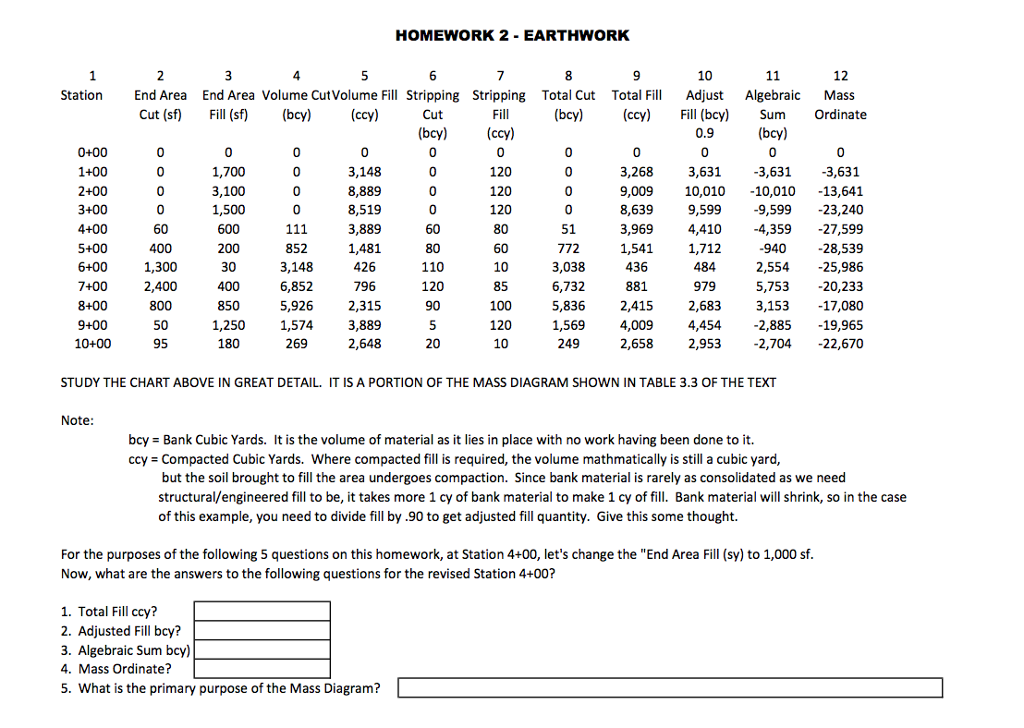# Answered Essay: STUDY THE CHART ABOVE IN GREAT DETAIL IT IS A PORTION OF THESTUDY THE CHART ABOVE IN GREAT DETAIL IT IS A PORTION OF THE MASS DIAGRAM SHOWN IN TABLE 3.3 OF THE TEXT For the purposes of the following 5 questions on this homework, at Station 4+00, let’s change the “End Area Fill (sy) to 1,000 sf. Now, what are the answers to the following questions for the revised Station 4+00? 1. Total Fill ccy? 2. Adjusted Fill bcy? 3. Algebraic Sum bcy) 4. Mass Ordinate? 5. What is the primary purpose of the Mass Diagram?

The change at Station 4+00 is:

End Area Fill = 1,000 sf.

Let us start calculating the revised values for Station 4+00 with the new value of End Area Fill i.e. 1,000 sf.

1. Station 4+00

2. End Area Cut (sf) = 60 sf [Same as existing condition, no change]

3. End Area Fill (sf) = 1,000 sf [Changed from existing condition, earlier it was 600 sf]

4. Volume Cut (bcy) = (Average Area Cut between Stations)*(Distance Between Stations)

= (Average Area Cut between Station 4+00 & 3+00)*(Distance between Station 4+00 & 3+00)

= [(60+0)/2] sf * (400-300) f = 3,000 cf = 3,000/27 cy = 111 cy [Same as existing condition]

[1 Cubic Yard (cy) = 27 Cubic Feet (cf); hence accordingly conversion has been done in the above step.

5. Volume Fill (ccy) = (Average Area Fill between Stations)*(Distance Between Stations)

= (Average Area Fill between Station 4+00 & 3+00)*(Distance between Station 4+00 & 3+00)

= [(1000+1500)/2] sf * (400-300) f = 1,25,000 cf = 1,25,000/27 cy = 4,630 cy [new value for ccy]

[1 Cubic Yard (cy) = 27 Cubic Feet (cf); hence accordingly conversion has been done in the above step.

6. Stripping Cut (bcy) = 60 [Same as existing condition]

7. Stripping Fill (ccy) = 80 [Same as existing condition]

8. Total Cut (bcy) = Volume Cut – Stripping Cut = 111 – 60 = 51 [Same as existing condition]

9. Total Fill (ccy) = Volume Fill + Stripping Fill = 4,630 + 80 = 4,710 [New Value for Total Fill ccy –> Answer for Q. 1]

10. Adjust Fill (bcy) = Total Fill (ccy)/Shrinkage Factor = 4710/0.9 = 5,233 bcy [Answer for Q. 2]

11. Algebric Sum (bcy) = Total Cut (bcy) – Adjusted Fill (bcy) = (51 – 5233) = -5,182 bcy [Answer for Q. 3]

12. Mass Ordinate at Current Station = Mass Ordinate at previous station + Algebric Sum at Current Station

= -23,240 + (-5,182) = -28,422 [Answer for Q. 4]

Hence, the final answers are:

1. Total Fill ccy = 4,710

2. Adjusted Fill bcy = 5,233

3. Algebric Sum bcy = -5,182

4. Mass Ordinate = -28,422

5. Primary Purpose of Mass Diagram –> Mass diagrams are used to determine the quantity of material moved and how far it can be moved. A mass diagram is a plot or graphical representation of the cumulative of Total Cut Volume & Total Fill Volume along an alignment. It also tells us about average haul, free haul and overhaul on a given segment of road.Calculate your paper price
Pages (550 words)
Approximate price: -

Help Me Write My Essay - Reasons:Best Online Essay Writing Service

We strive to give our customers the best online essay writing experience. We Make sure essays are submitted on time and all the instructions are followed.Our Writers are Experienced and Professional

Our essay writing service is founded on professional writers who are on stand by to help you any time.Free Revision Fo all Essays

Sometimes you may require our writers to add on a point to make your essay as customised as possible, we will give you unlimited times to do this. And we will do it for free.Timely Essay(s)

We understand the frustrations that comes with late essays and our writers are extra careful to not violate this term. Our support team is always engauging our writers to help you have your essay ahead of time.Customised Essays &100% Confidential

Our Online writing Service has zero torelance for plagiarised papers. We have plagiarism checking tool that generate plagiarism reports just to make sure you are satisfied.Our agents are ready to help you around the clock. Please feel free to reach out and enquire about anything.

Try it now!

## Calculate the price of your order

Total price:
\$0.00

How it works?

Follow these simple steps to get your paper doneFill in the order form and provide all details of your assignment.Proceed with the payment

Choose the payment system that suits you most.Receive the final file

Once your paper is ready, we will email it to you.

HOW OUR ONLINE ESSAY WRITING SERVICE WORKS

Let us write that nagging essay.## Submit Your Essay/Homework Instructions

By clicking on the "PLACE ORDER" button, tell us your requires. Be precise for an accurate customised essay. You may also upload any reading materials where applicable.Pick A & Writer

Our ordering form will provide you with a list of writers and their feedbacks. At step 2, its time select a writer. Our online agents are on stand by to help you just in case.Editing (OUR PART)

At this stage, our editor will go through your essay and make sure your writer did meet all the instructions.